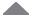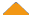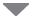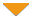Run Code  | API  | Code Wall  | Users  | Misc  | Feedback  | Login  | Theme  | Privacy  | Patreon

 run  | edit  | history  | help0## Title of this code

require(stats) plot(cars) lines(lowess(cars))

plot(sin, -pi, 2*pi) # see ?plot.function

## Discrete Distribution Plot:

plot(table(rpois(100, 5)), type = "h", col = "red", lwd = 10, main = "rpois(100, lambda = 5)")

## Simple quantiles/ECDF, see ecdf() {library(stats)} for a better one:

plot(x <- sort(rnorm(47)), type = "s", main = "plot(x, type = \"s\")") points(x, cex = .5, col = "dark red")

by  psrpsrpsrpsr, 4 years ago

x=c(5,2,7,8,3) median(x) mod(x) var(x)

by  Cemile Yeşilkaya, 11 months ago